Science, Maths & Technology

### Become an OU studentEveryday maths 1 (Wales)

Start this free course now. Just create an account and sign in. Enrol and complete the course for a free statement of participation or digital badge if available.

# 3.1 Instruments of measure

Scales show you how much something weighs. Digital scales show the weight as a display of numbers. Other scales have a dial or line of numbers and you have to read the weight from this.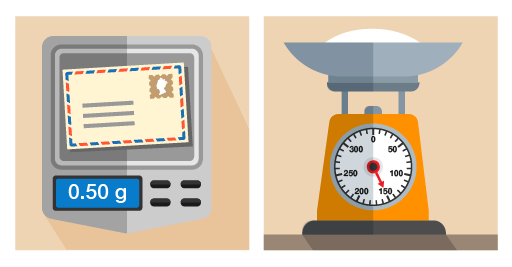Figure 20 Using different scales for different objects

You’ll notice that on the right-hand set of scales in the picture above, the needle points to 150 g. If you use scales like this, you need to know the divisions marked on the scales. You might have to count the marks between numbers.

## Example: Identifying weights on scales

What is the weight of the flour in these scales?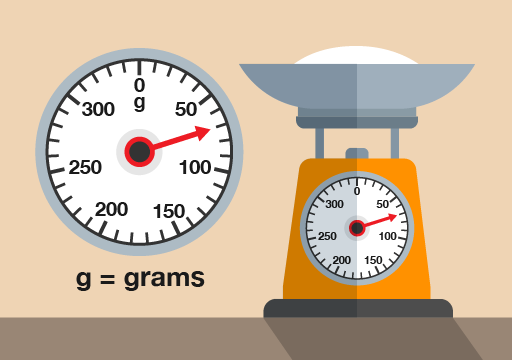Figure 21 Weighing flour

(Note that scales like this are calibrated to weigh only the flour inside the bowl – the weight on the scales is just the flour, not the flour and the bowl.)

### Method

There are four marks between 50 g and 100 g, each representing another 10 g. So the marks represent 60 g, 70 g, 80 g and 90 g. The needle is level with the second mark, so the weight is 70 g.

Now try the following activity. Remember to check your answers once you have completed the questions.

1. How many grams of sugar are on the scales in the picture below?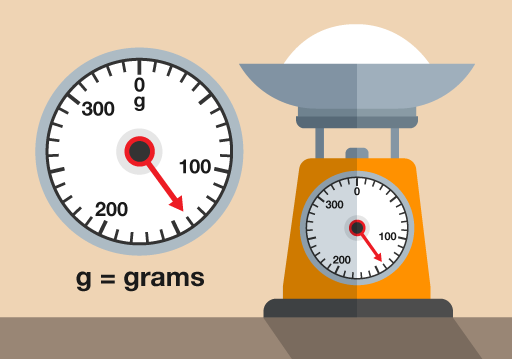Figure 22 Weighing sugar
2. What is this person’s weight in kilograms?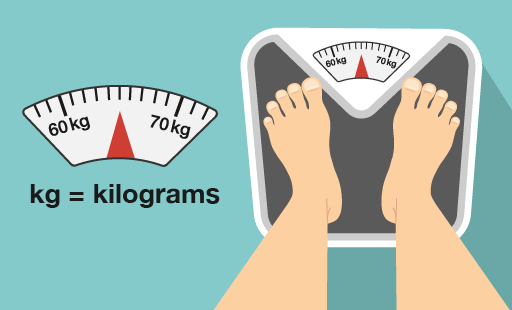Figure 23 Weighing a person
3. How much does the letter weigh?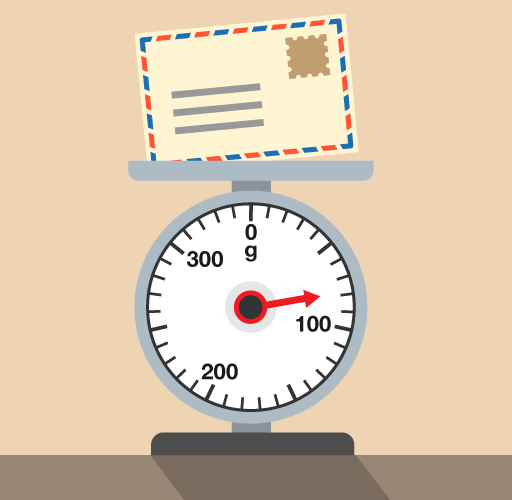Figure 24 Weighing a letter